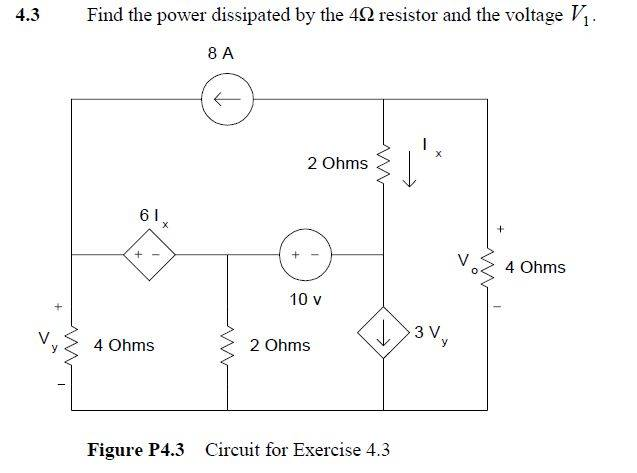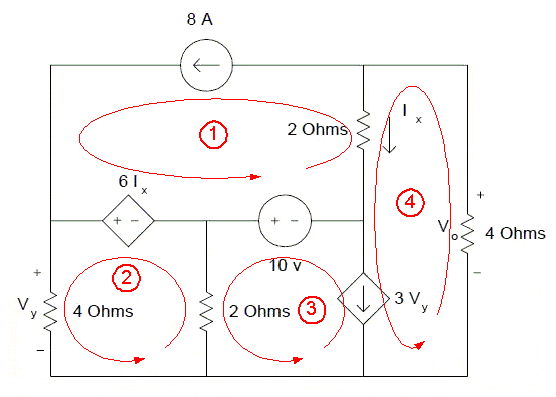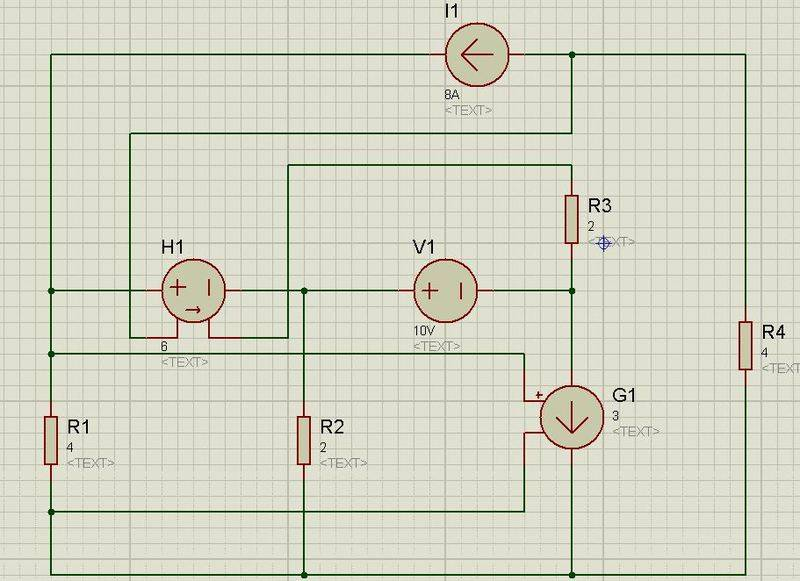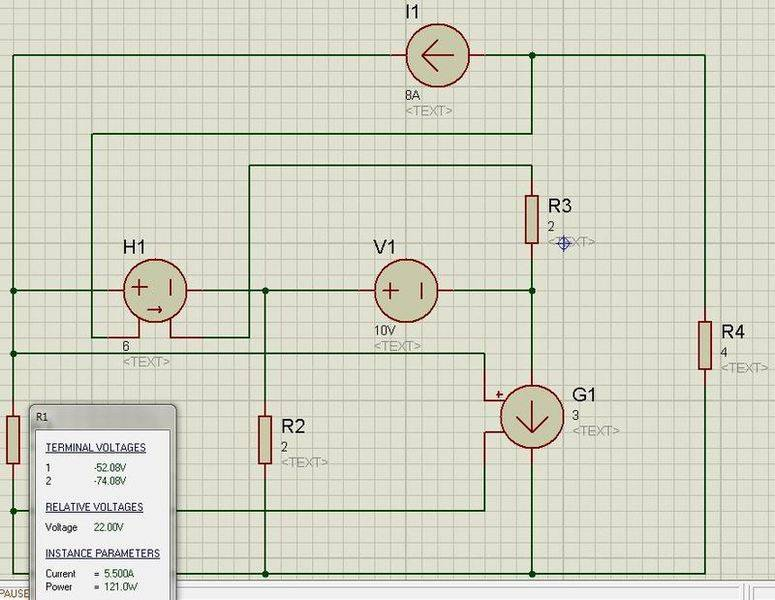# Mesh Current Method with Dependent Sources

## Homework StatementV=I*R

## The Attempt at a Solution

I started writing the equations for each loop.

Loop 1 -> I1=8A
Loop 2 -> -6Ix + 4I2 + 2(I2-I3) =0 and Ix=I4-I2
Loop 3 -> I dont know how to approach the dependent current source here.
Loop 4 -> Same here

What I ask is are these equations for loop 1 and 2 is true? If they are not, whats wrong? And how can I write the equations for loop 3 and 4?

gneill
Mentor
Hi warden13, Welcome to Physics Forums.

Mesh analysis with current supplies can be a tad tricky at times. If a supply is shared by only one loop then it defines that loop's mesh current and you declare that current "solved". This is what you've done with your loop 1 by setting ##I_1 = 8 A##.

When a current source straddles two loops, as the ##3V_y## source does, you can introduce another loop variable to represent the potential change across that current supply. Use this new unknown in your loop KVL equations. The current value of the supply is then used to write another equation relating the currents of the two loops. In fact, as you write your loop equations you should end up collecting enough of these "auxiliary equations" relating current supplies to mesh currents to be able to reduce the number of unknowns to match the number of loops.

Here's your circuit with loop numbers and mesh currents indicated:Suppose you call the potential change across the controlled current Vx, assumed to be a potential rise in the direction of the current. Then how might you write your loop 3 equation?

#### Attachments

If I got what you are saying, then loop 3 equations is:

-10 + 2(I3-I2) + Vx = 0

and loop 4 is:

2(I4-I1) - Vx + 4I4 = 0

correct?

But how do i find the Vx?

Last edited:
gneill
Mentor
If I got what you are saying, then loop 3 equations is:

-10 + 2(I3-I2) + Vx = 0

and loop 4 is:

2(I4-I1) - Vx + 4I4 = 0

correct?

But how do i find the Vx?

Yes, that looks good. Now, there are relationships imposed by the current sources which relate the mesh currents. You should be able to replace I4 with a combination of other mesh currents. That'll eliminate I4 from your equation set, leaving I2, I3, and Vx as unknowns, and you should have three equations...

I couldnt replace I4, can you tell me how to replace it?

gneill
Mentor
I couldnt replace I4, can you tell me how to replace it?

Well, there's a small chain of relationships involved.

From loop 2 : ##V_y = 4 I_2##

From loop 4: ##3 V_y = I_4 - I_3##

Go from there.

So i found the following equations:

Code:
eq1=((-6*(((12*i2)+i3)-i2)) + (4*i2) + (2*(i2-i3)));

eq2=((-10) + 2*(i3-i2) + vx );

eq3=((2*((((12*i2)+i3))-(8))) + (-vx) + (4*(((12*i2)+i3))));

When i solve it with matlab, the results are:

solutions_i2 = 13/5

solutions_i3 = -39/2

solutions_vx = 271/5

But the problem is, i am not so sure about the answers. I simulated the circuit it pspice schematics, but found different results. I wonder if these equations are true.

gneill
Mentor
Well, something's gone awry with your equations since your post #3Your results don't match what I found, and they should match a correct simulation, too.

Perhaps you can spell out your workings in more detail.

Edit:
I took a weed-whacker to the forest of parentheses in your expressions and boiled them down to:

1) ##-60i_2 - 8 i_3##
2) ##-10 + 2 i_3 - 2 i_2 + Vx##
3) ##72 i_2 + 6 i_3 - 16 - Vx##

Note that I refer to them as expressions and not as equations; for them to be equations there would have to be an equals sign in there somewhere.

Assuming that each of them should really be set equal to zero, then "equations" 2 and 3 look okay to me, but #1 has a problem with its ##i_2## term.

Last edited:
Ok your expressions and mine are same. But that means I still get different results than im supposed to get.

For instance, when i simulate the circuit, i find the i2 = 5.5A

But in our equations, 13/5=2.6

Code:
You can solve them here:
[url]http://www.numberempire.com/equationsolver.php[/url]
in this format:

((-6*(((12*i2)+i3)-i2))+(4*i2)+(2*(i2-i3))),((-10)+2*(i3-i2)+vx),((2*((((12*i2)+i3))-(8)))+(-vx)+(4*(((12*i2)+i3))))

and variables:

i2,i3,vx

My simulation:The values of R1Sorry for big images,long post.

gneill
Mentor
Ok your expressions and mine are same. But that means I still get different results than im supposed to get.

For instance, when i simulate the circuit, i find the i2 = 5.5A

But in our equations, 13/5=2.6

No, not all of our equations are the same. Our first equations (Loop 2) are different.